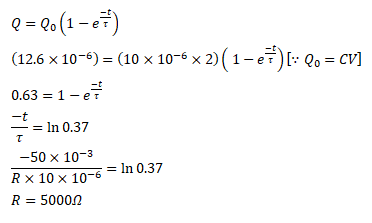# A capacitor of capacitance 10 μF is connected to

Question:

A capacitor of capacitance $10 \mu \mathrm{F}$ is connected to a battery of emf $2 \mathrm{~V}$. It is found that it takes $50 \mathrm{~ms}$ for the charge on the capacitor to become $12.6 \mu \mathrm{C}$. Find the resistance of the circuit.

Solution: